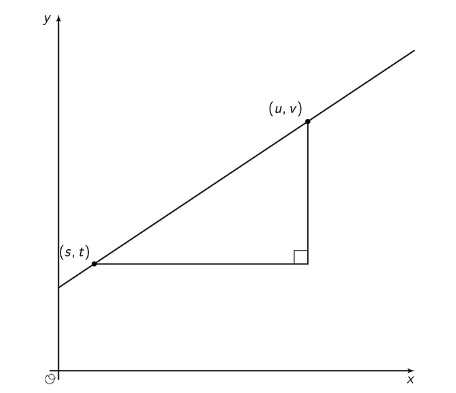PSABM6V6 - Student Facing Task---Acc7.5 Lesson 6 Representations of Linear Relationships (8.EE.B, 8.EE.B.6)
Part A)

Which glass will hold the most water?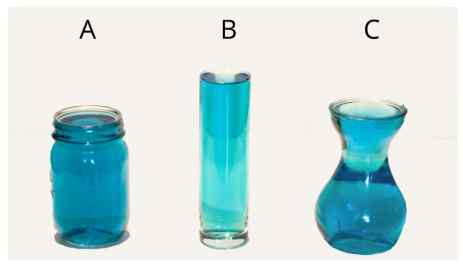Part B)

Which glass will hold the least water?

Part A)

Record the data from your teacher's demonstration in the table. (You may not need all the rows.)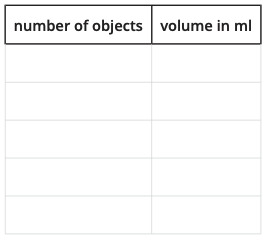Copy the table by using the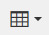button.

Part B)

What is the volume, V, in the cylinder after you add x objects? Explain your reasoning.

Part C)

If you wanted to make the water reach the highest mark on the cylinder, how many objects would you need?

Part D)

Plot and label points that show your measurements from the experiment.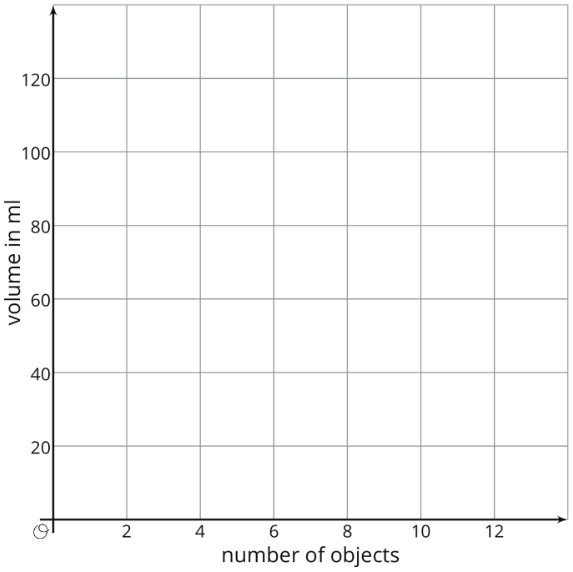Copy the coordinate plane on a piece of paper, then plot your points. Take a picture and upload it using the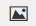button. If you cannot, write "Graph is on paper."

Part E)

The points should fall on a line. Use a ruler to graph this line.

Draw the line on the graph you just constructed. Then take another picture and upload it using thebutton. If you cannot, write "Graph is on paper."

Part F)

Compute the slope of the line. What does the slope mean in this situation?

Part G)

What is the vertical intercept? What does the vertical intercept mean in this situation?

Part A)

A situation is represented by the equationInvent a story for this situation.

Part B)

Graph the equation.

Draw your graph on paper, take a picture, and upload it using the image upload icon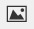If you do not have the ability to upload an image of your work, type "Graph is on paper."

Part C)

What do theand the 5 represent in your situation?

Part D)

Where do you see theand 5 on the graph?

Part A)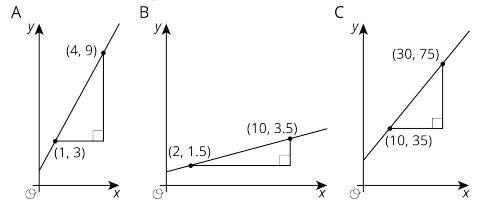For each graph, record: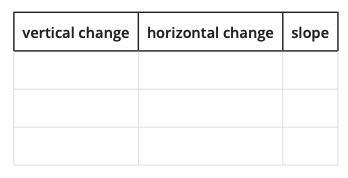Create and fill in the table using the table button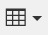Part B)

Describe a procedure for finding the slope between any two points on a line.